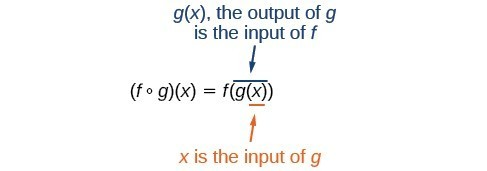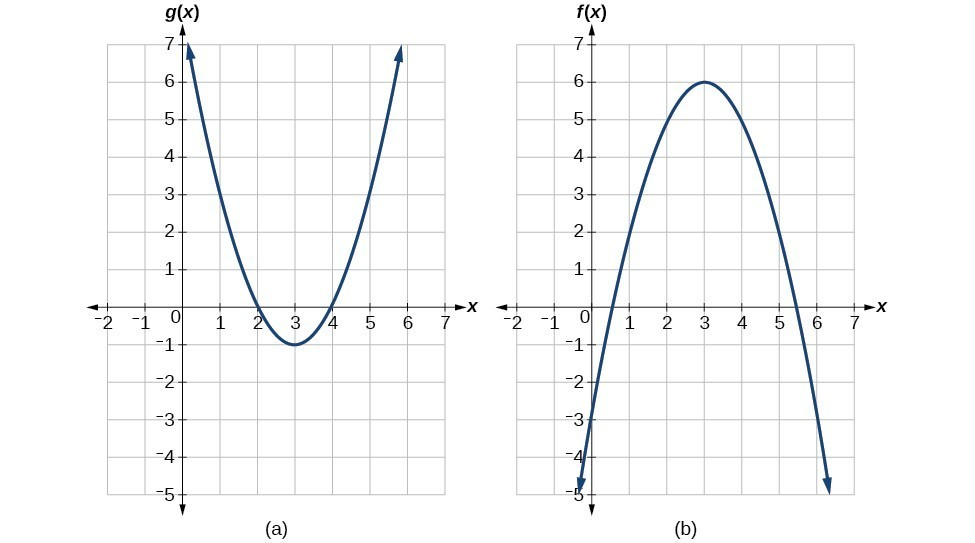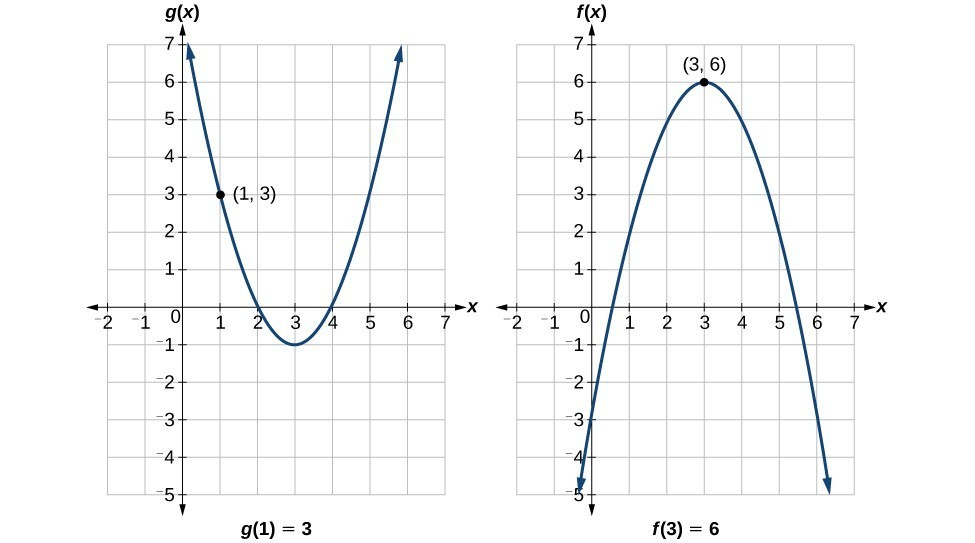## Compositions of Functions

### Learning Outcomes

• Combine functions using algebraic operations.
• Create a new function by composition of functions.
• Evaluate composite functions.
• Find the domain of a composite function.
• Decompose a composite function into its component functions.

Suppose we want to calculate how much it costs to heat a house on a particular day of the year. The cost to heat a house will depend on the average daily temperature, and in turn, the average daily temperature depends on the particular day of the year. Notice how we have just defined two relationships: The cost depends on the temperature, and the temperature depends on the day.Figure 1

Using descriptive variables, we can notate these two functions. The function $C\left(T\right)$ gives the cost $C$ of heating a house for a given average daily temperature in $T$ degrees Celsius. The function $T\left(d\right)$ gives the average daily temperature on day $d$ of the year. For any given day, $\text{Cost}=C\left(T\left(d\right)\right)$ means that the cost depends on the temperature, which in turns depends on the day of the year. Thus, we can evaluate the cost function at the temperature $T\left(d\right)$. For example, we could evaluate $T\left(5\right)$ to determine the average daily temperature on the 5th day of the year. Then, we could evaluate the cost function at that temperature. We would write $C\left(T\left(5\right)\right)$.

By combining these two relationships into one function, we have performed function composition, which is the focus of this section.

## Compositions of Functions

Function composition is only one way to combine existing functions. Another way is to carry out the usual algebraic operations on functions, such as addition, subtraction, multiplication and division. We do this by performing the operations with the function outputs, defining the result as the output of our new function.

Suppose we need to add two columns of numbers that represent a husband and wife’s separate annual incomes over a period of years, with the result being their total household income. We want to do this for every year, adding only that year’s incomes and then collecting all the data in a new column. If $w\left(y\right)$ is the wife’s income and $h\left(y\right)$ is the husband’s income in year $y$, and we want $T$ to represent the total income, then we can define a new function.

$T\left(y\right)=h\left(y\right)+w\left(y\right)$

If this holds true for every year, then we can focus on the relation between the functions without reference to a year and write

$T=h+w$

Just as for this sum of two functions, we can define difference, product, and ratio functions for any pair of functions that have the same kinds of inputs (not necessarily numbers) and also the same kinds of outputs (which do have to be numbers so that the usual operations of algebra can apply to them, and which also must have the same units or no units when we add and subtract). In this way, we can think of adding, subtracting, multiplying, and dividing functions.

For two functions $f\left(x\right)$ and $g\left(x\right)$ with real number outputs, we define new functions $f+g,f-g,f\cdot{g}$, and $\dfrac{f}{g}$ by the relations

\begin{align}{}\left(f+g\right)\left(x\right)&=f\left(x\right)+g\left(x\right) \\[2mm] \left(f-g\right)\left(x\right)&=f\left(x\right)-g\left(x\right) \\[2mm] \text{ }\left(f\cdot{g}\right)\left(x\right)&=f\left(x\right)\cdot{g}\left(x\right) \\[2mm] \text{ }\left(\dfrac{f}{g}\right)\left(x\right)&=\dfrac{f\left(x\right)}{g\left(x\right)}\\& \end{align}

### Example: Performing Algebraic Operations on Functions

Find and simplify the functions $\left(g-f\right)\left(x\right)$ and $\left(\dfrac{g}{f}\right)\left(x\right)$, given $f\left(x\right)=x - 1$ and $g\left(x\right)={x}^{2}-1$. Give the domain of your result. Are they the same function?

### Try It

Find and simplify the functions $\left(f\cdot{g}\right)\left(x\right)$ and $\left(f-g\right)\left(x\right)$.

$f\left(x\right)=x - 1\text{ and }g\left(x\right)={x}^{2}-1$

Are they the same function?

### try it

Let’s explore what happens to the graphs of functions that are combined using algebraic operations. Use an online graphing tool to graph the following functions:

1. $f(x) = x^2+3x-4$
2. $g(x) = \dfrac{1}{x-1}$

Now, enter $h(x) = f(x)+g(x)$ into the next line.

Evaluate $h(1)$, why do you think you get this result?

### Create a New Function Using a Composition

Performing algebraic operations on functions combines them into a new function, but we can also create functions by composing functions. When we wanted to compute a heating cost from a day of the year, we created a new function that takes a day as input and yields a cost as output. The process of combining functions so that the output of one function becomes the input of another is known as a composition of functions. The resulting function is known as a composite function. We represent this combination by the following notation:

$\left(f\circ g\right)\left(x\right)=f\left(g\left(x\right)\right)$

We read the left-hand side as $f$ composed with $g$ at $x,''$ and the right-hand side as $f$ of $g$ of $x.''$ The two sides of the equation have the same mathematical meaning and are equal. The open circle symbol $\circ$ is called the composition operator. We use this operator mainly when we wish to emphasize the relationship between the functions themselves without referring to any particular input value. Composition is a binary operation that takes two functions and forms a new function, much as addition or multiplication takes two numbers and gives a new number. However, it is important not to confuse function composition with multiplication because, as we learned above, in most cases $f\left(g\left(x\right)\right)\ne f\left(x\right)g\left(x\right)$.

It is also important to understand the order of operations in evaluating a composite function. We follow the usual convention with parentheses by starting with the innermost parentheses first, and then working to the outside. In the equation above, the function $g$ takes the input $x$ first and yields an output $g\left(x\right)$. Then the function $f$ takes $g\left(x\right)$ as an input and yields an output $f\left(g\left(x\right)\right)$.Figure 2

In general $f\circ g$ and $g\circ f$ are different functions. In other words in many cases $f\left(g\left(x\right)\right)\ne g\left(f\left(x\right)\right)$ for all $x$.

For example if $f\left(x\right)={x}^{2}$ and $g\left(x\right)=x+2$, then

\begin{align}f\left(g\left(x\right)\right)&=f\left(x+2\right) \\[2mm] &={\left(x+2\right)}^{2} \\[2mm] &={x}^{2}+4x+4\hfill \end{align}

but

\begin{align}g\left(f\left(x\right)\right)&=g\left({x}^{2}\right) \\[2mm] \text{ }&={x}^{2}+2\hfill \end{align}

These expressions are not equal for all values of $x$, so the two functions are not equal. It is irrelevant that the expressions happen to be equal for the single input value $x=-\frac{1}{2}$.

Note that the range of the inside function (the first function to be evaluated) needs to be within the domain of the outside function. Less formally, the composition has to make sense in terms of inputs and outputs. Additionally, in applied settings, function composition usually only makes sense in one specific order.

### A General Note: Composition of Functions

When the output of one function is used as the input of another, we call the entire operation a composition of functions. For any input $x$ and functions $f$ and $g$, this action defines a composite function, which we write as $f\circ g$ such that

$\left(f\circ g\right)\left(x\right)=f\left(g\left(x\right)\right)$

The domain of the composite function $f\circ g$ is all $x$ such that $x$ is in the domain of $g$ and $g\left(x\right)$ is in the domain of $f$.

It is important to realize that the product of functions $fg$ is not the same as the function composition $f\left(g\left(x\right)\right)$, because, in general, $f\left(x\right)g\left(x\right)\ne f\left(g\left(x\right)\right)$.

### Example: Define a Composition of Functions

Using the functions provided, find $f\left(g\left(x\right)\right)$ and $g\left(f\left(x\right)\right)$.
$f\left(x\right)=2x+1\\g\left(x\right)=3-x$

### try it

Now we will explore the graph of a composition of functions. Use an online graphing tool to graph the following functions:

1. $f(x) = x^2+3x-4$
2. $g(x) = \dfrac{1}{x-1}$

Now define a new function:

$h(x) = f(f(x))$

Now zoom in (use the plus sign in the upper right-hand corner of the graph) on the point $(0.23607,-3.236)$, notice that both graphs pass through this point. Continue this pattern for a few more iterations: define a new function that contains another composition of $f(x)$ with itself. For example: $p(x) = f(f(f(x)))$, and so on. Continue to use the zoom as you iterate.

The point $(0.23607,-3.236)$ is called a fixed point of the function $f(x)=x^2+3x-4$. Fixed points are used in mathematical applications such as the page rank algorithm that Google uses to generate internet search results. Read more about fixed points here, and page rank here.

### Example: Interpreting Composite Functions

The function $c\left(s\right)$ gives the number of calories burned completing $s$ sit-ups, and $s\left(t\right)$ gives the number of sit-ups a person can complete in $t$ minutes. Interpret $c\left(s\left(3\right)\right)$.

### Example: Investigating the Order of Function Composition

Suppose $f\left(x\right)$ gives miles that can be driven in $x$ hours and $g\left(y\right)$ gives the gallons of gas used in driving $y$ miles. Which of these expressions is meaningful: $f\left(g\left(y\right)\right)$ or $g\left(f\left(x\right)\right)?$

### Q & A

Are there any situations where $f\left(g\left(y\right)\right)$ and $g\left(f\left(x\right)\right)$ would both be meaningful or useful expressions?

Yes. For many pure mathematical functions, both compositions make sense, even though they usually produce different new functions. In real-world problems, functions whose inputs and outputs have the same units also may give compositions that are meaningful in either order.

### Try It

The gravitational force on a planet a distance r from the sun is given by the function $G\left(r\right)$. The acceleration of a planet subjected to any force $F$ is given by the function $a\left(F\right)$. Form a meaningful composition of these two functions, and explain what it means.

## Evaluate a Composition of Functions

Once we compose a new function from two existing functions, we need to be able to evaluate it for any input in its domain. We will do this with specific numerical inputs for functions expressed as tables, graphs, and formulas and with variables as inputs to functions expressed as formulas. In each case we evaluate the inner function using the starting input and then use the inner function’s output as the input for the outer function.

### Evaluating Composite Functions Using Tables

When working with functions given as tables, we read input and output values from the table entries and always work from the inside to the outside. We evaluate the inside function first and then use the output of the inside function as the input to the outside function.

### Example: Using a Table to Evaluate a Composite Function

Using the table below, evaluate $f\left(g\left(3\right)\right)$ and $g\left(f\left(3\right)\right)$.

$x$ $f\left(x\right)$ $g\left(x\right)$
1 6 3
2 8 5
3 3 2
4 1 7

### Try It

Using the table below, evaluate $f\left(g\left(1\right)\right)$ and $g\left(f\left(4\right)\right)$.

$x$ $f\left(x\right)$ $g\left(x\right)$
1 6 3
2 8 5
3 3 2
4 1 7

### Evaluating Composite Functions Using Graphs

When we are given individual functions as graphs, the procedure for evaluating composite functions is similar to the process we use for evaluating tables. We read the input and output values, but this time, from the $x\text{-}$ and $y\text{-}$ axes of the graphs.

### How To: Given a composite function and graphs of its individual functions, evaluate it using the information provided by the graphs.

1. Locate the given input to the inner function on the $x\text{-}$ axis of its graph.
2. Read off the output of the inner function from the $y\text{-}$ axis of its graph.
3. Locate the inner function output on the $x\text{-}$ axis of the graph of the outer function.
4. Read the output of the outer function from the $y\text{-}$ axis of its graph. This is the output of the composite function.

### Example: Using a Graph to Evaluate a Composite Function

Using the graphs below, evaluate $f\left(g\left(1\right)\right)$.### Try It

Using the graphs below, evaluate $g\left(f\left(2\right)\right)$.### Evaluating Composite Functions Using Formulas

When evaluating a composite function where we have either created or been given formulas, the rule of working from the inside out remains the same. The input value to the outer function will be the output of the inner function, which may be a numerical value, a variable name, or a more complicated expression.

While we can compose the functions for each individual input value, it is sometimes helpful to find a single formula that will calculate the result of a composition $f\left(g\left(x\right)\right)$. To do this, we will extend our idea of function evaluation. Recall that, when we evaluate a function like $f\left(t\right)={t}^{2}-t$, we substitute the value inside the parentheses into the formula wherever we see the input variable.

### How To: Given a formula for a composite function, evaluate the function.

1. Evaluate the inside function using the input value or variable provided.
2. Use the resulting output as the input to the outside function.

### Example: Evaluating a Composition of Functions Expressed as Formulas with a Numerical Input

Given $f\left(t\right)={t}^{2}-{t}$ and $h\left(x\right)=3x+2$, evaluate $f\left(h\left(1\right)\right)$.

### Try It

Given $f\left(t\right)={t}^{2}-t$ and $h\left(x\right)=3x+2$, evaluate

A) $h\left(f\left(2\right)\right)$

B) $h\left(f\left(-2\right)\right)$

## Domain of a Composition

As we discussed previously, the domain of a composite function such as $f\circ g$ is dependent on the domain of $g$ and the domain of $f$. It is important to know when we can apply a composite function and when we cannot, that is, to know the domain of a function such as $f\circ g$. Let us assume we know the domains of the functions $f$ and $g$ separately. If we write the composite function for an input $x$ as $f\left(g\left(x\right)\right)$, we can see right away that $x$ must be a member of the domain of $g$ in order for the expression to be meaningful, because otherwise we cannot complete the inner function evaluation. However, we also see that $g\left(x\right)$ must be a member of the domain of $f$, otherwise the second function evaluation in $f\left(g\left(x\right)\right)$ cannot be completed, and the expression is still undefined. Thus the domain of $f\circ g$ consists of only those inputs in the domain of $g$ that produce outputs from $g$ belonging to the domain of $f$. Note that the domain of $f$ composed with $g$ is the set of all $x$ such that $x$ is in the domain of $g$ and $g\left(x\right)$ is in the domain of $f$.

### A General Note: Domain of a Composite Function

The domain of a composite function $f\left(g\left(x\right)\right)$ is the set of those inputs $x$ in the domain of $g$ for which $g\left(x\right)$ is in the domain of $f$.

### How To: Given a function composition $f\left(g\left(x\right)\right)$, determine its domain.

1. Find the domain of g.
2. Find the domain of f.
3. Find those inputs, $x$, in the domain of $g$ for which $g(x)$ is in the domain of $f$. That is, exclude those inputs, $x$, from the domain of $g$ for which $g(x)$ is not in the domain of $f$. The resulting set is the domain of $f\circ g$.

### Example: Finding the Domain of a Composite Function

Find the domain of

$\left(f\circ g\right)\left(x\right)\text{ where}f\left(x\right)=\dfrac{5}{x - 1}\text{ and }g\left(x\right)=\dfrac{4}{3x - 2}$

### Example: Finding the Domain of a Composite Function Involving Radicals

Find the domain of

$\left(f\circ g\right)\left(x\right)\text{ where}f\left(x\right)=\sqrt{x+2}\text{ and }g\left(x\right)=\sqrt{3-x}$

### Try It

Find the domain of

$\left(f\circ g\right)\left(x\right)\text{ where}f\left(x\right)=\dfrac{1}{x - 2}\text{ and }g\left(x\right)=\sqrt{x+4}$

### try it

We can use graphs to visualize the domain that results from a composition of two functions.
Graph the two functions below with an online graphing tool.

1. $f(x)=\sqrt{3-x}$
2. $g(t) = \sqrt{x+4}$

Next, create a new function, $h(x) = g(f(x))$.  Based on the graph, what is the domain of this function? Explain why $g(f(x))$ and $f(x)$ have the same domain.

Now define another composition, $p(x) = f(g(x)$.  What is the domain of this function? Explain why you can evaluate $g(10)$, but not $p(10)$.

### Decompose a Composite Function

In some cases, it is necessary to decompose a complicated function. In other words, we can write it as a composition of two simpler functions. There is almost always more than one way to decompose a composite function, so we may choose the decomposition that appears to be most obvious.

### Example: Decomposing a Function

Write $f\left(x\right)=\sqrt{5-{x}^{2}}$ as the composition of two functions.

### Try It

Write $f\left(x\right)=\dfrac{4}{3-\sqrt{4+{x}^{2}}}$ as the composition of two functions.

## Key Equation

 Composite function $\left(f\circ g\right)\left(x\right)=f\left(g\left(x\right)\right)$

## Key Concepts

• We can perform algebraic operations on functions.
• When functions are combined, the output of the first (inner) function becomes the input of the second (outer) function.
• The function produced by combining two functions is a composite function.
• The order of function composition must be considered when interpreting the meaning of composite functions.
• A composite function can be evaluated by evaluating the inner function using the given input value and then evaluating the outer function taking as its input the output of the inner function.
• A composite function can be evaluated from a table.
• A composite function can be evaluated from a graph.
• A composite function can be evaluated from a formula.
• The domain of a composite function consists of those inputs in the domain of the inner function that correspond to outputs of the inner function that are in the domain of the outer function.
• Just as functions can be combined to form a composite function, composite functions can be decomposed into simpler functions.
• Functions can often be decomposed in more than one way.

## Glossary

composite function
the new function formed by function composition, when the output of one function is used as the input of another